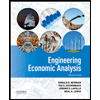# 3. Consider a competitive firm with total costs given by TC(q) 10010q+ q? The firm faces a market price p = 50 (a) Write expressions for total revenue TR and marginal revenue MR as functions of output q (b) Write expressions for average total cost ATC, average variable cost AVC, and marginal cost MC as functions of output q (c) For what value of output is ATC minimized? 1 (d) Find the profit maximizing level of output q. At this level of output, what are TR, TC, ATC, and *? (e) Graph the ATC, AVC, MC, and MR curves in a single graph, and indicate the profit maximizing level of output. If there are profits, shade the region corre- sponding to profit and label it. (f) If fixed costs increase from 100 to 500, what happens to the profit maximizing level of output, TR, TC, and ? (g) If fixed costs increase from 100 to 500, should the firm continue to operate in the short-run? What about the long-run?

Question

Expert Solution

### Want to see the full answer?

Check out a sample Q&A hereStudents who’ve seen this question also like:ENGR.ECONOMIC ANALYSIS
14th Edition
ISBN: 9780190931919
Author: NEWNAN
Publisher: Oxford University Press